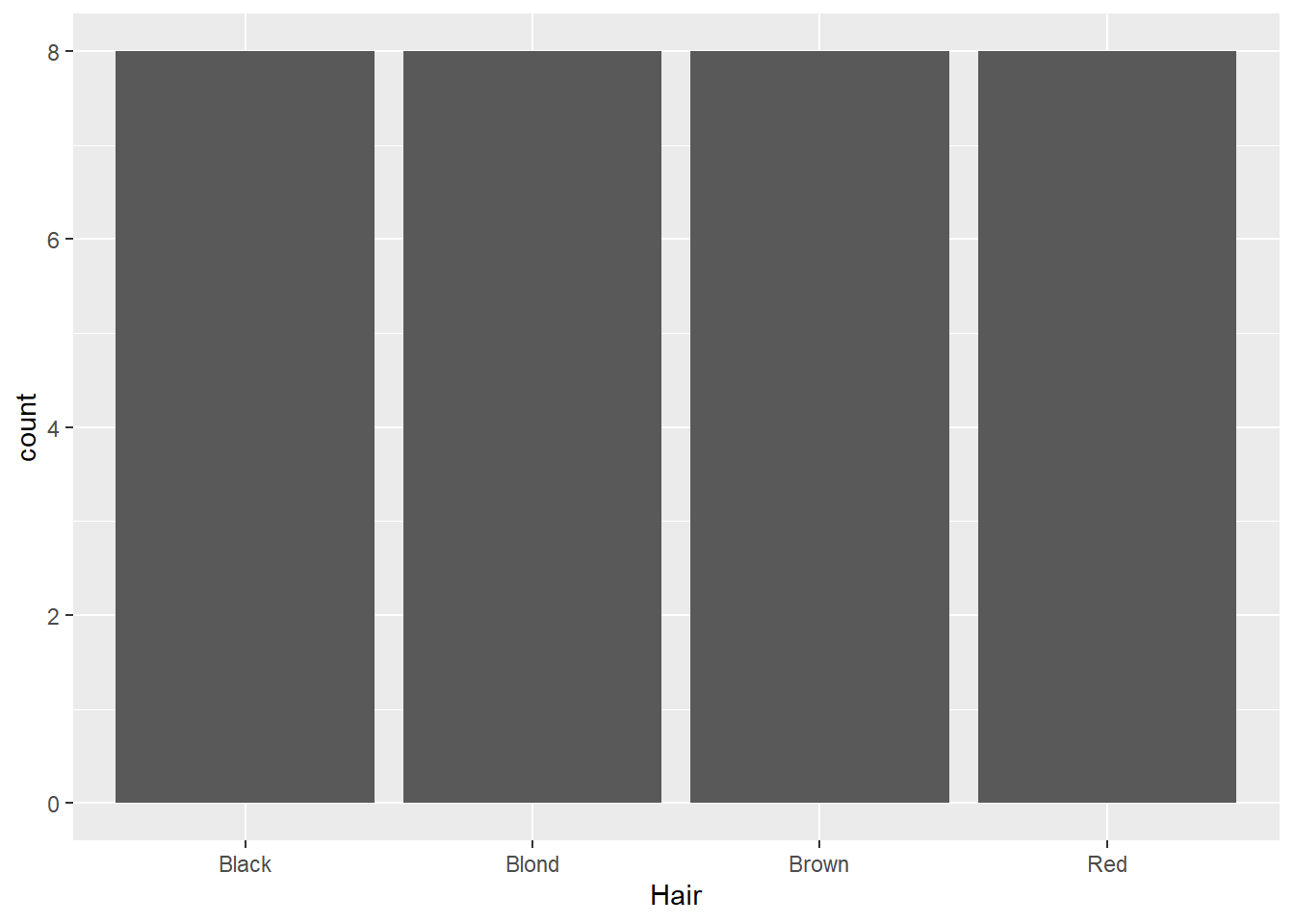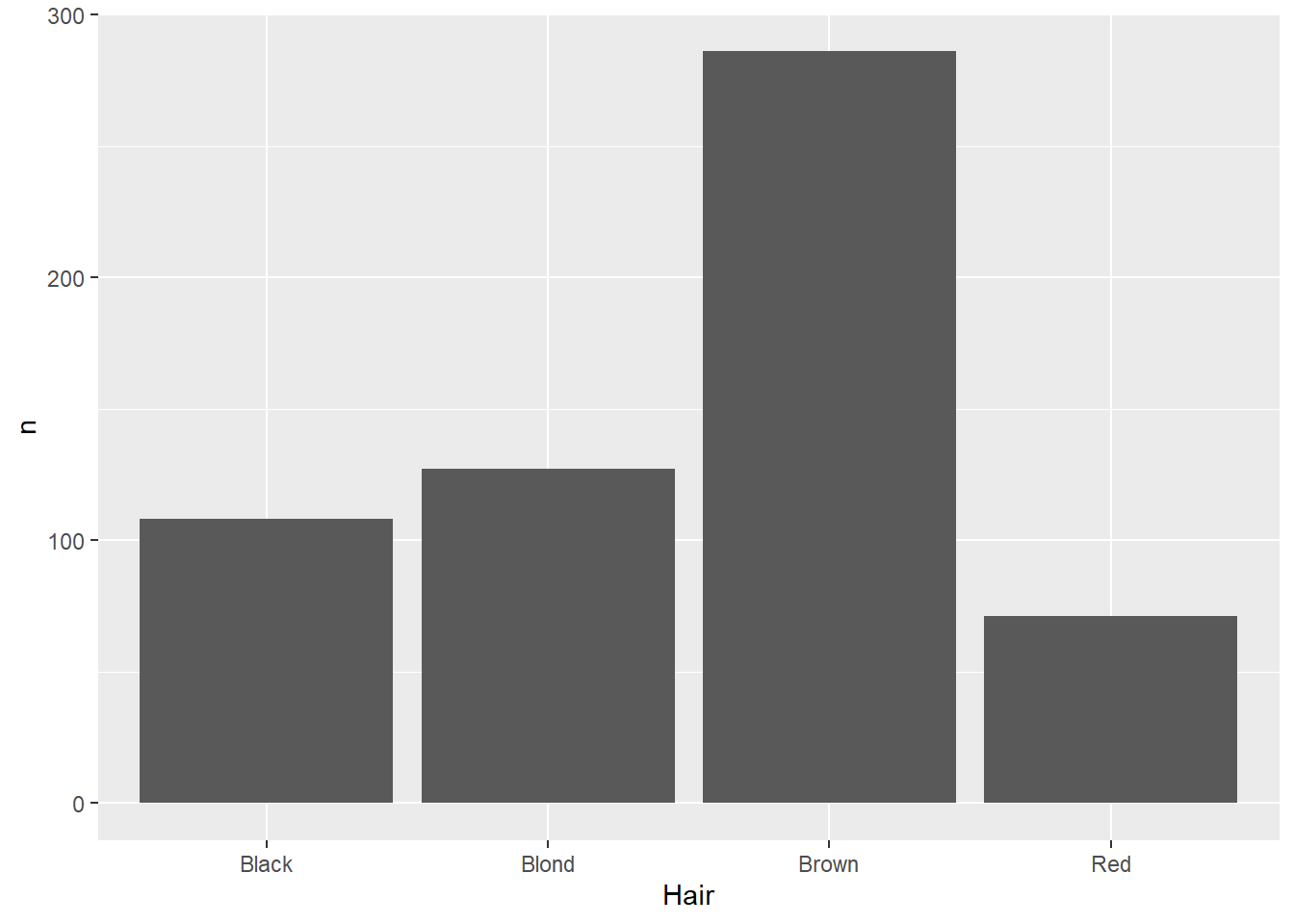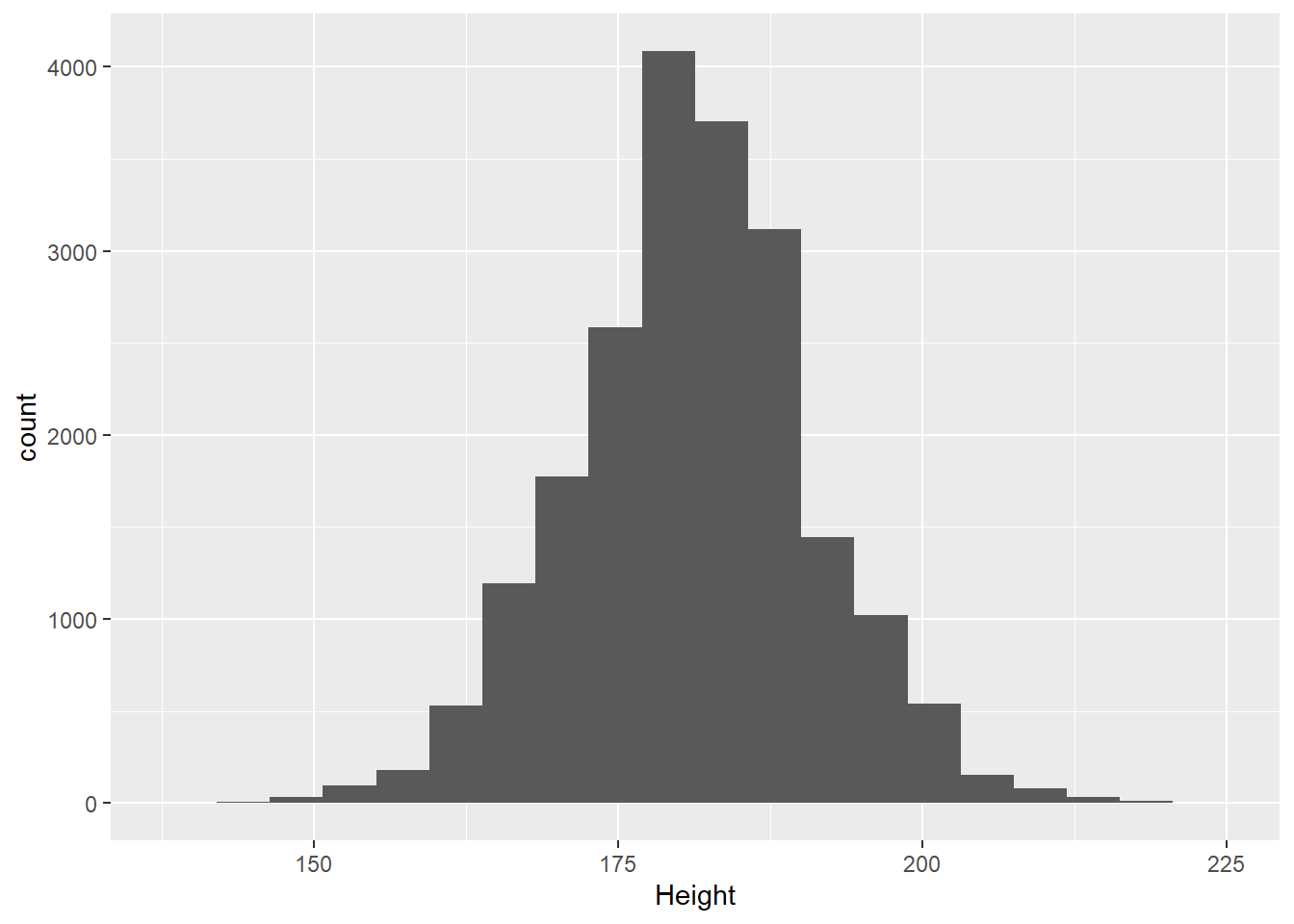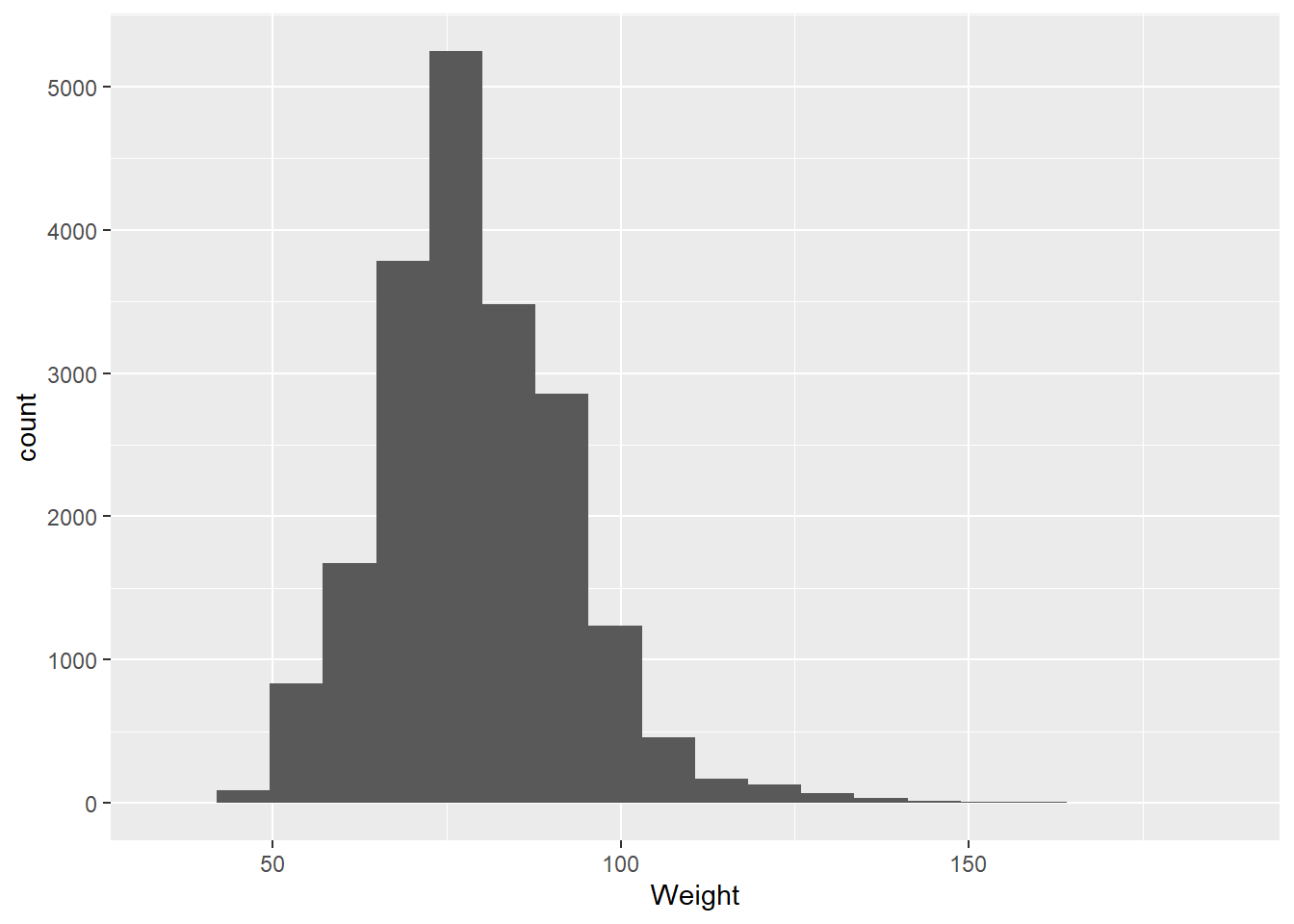# 2Looking for Patterns in One Variable

There’s no right or wrong way to do EDA, but many often start simply by exploring patterns in single variables. This is called univariate analysis. We do this

#### Nominal/Ordinal

What we’re looking for here are relative proportions. Does some categories stand out from the others? We’ve seen the geom_bar geometry be used for this. But what if the counts are already in the data? For example, look at the `HairEyeColor` dataset from the modeldata package:

``````hairEye<-as_tibble(HairEyeColor)
hairEye``````
``````# A tibble: 32 × 4
Hair  Eye   Sex       n
<chr> <chr> <chr> <dbl>
1 Black Brown Male     32
2 Brown Brown Male     53
3 Red   Brown Male     10
4 Blond Brown Male      3
5 Black Blue  Male     11
6 Brown Blue  Male     50
7 Red   Blue  Male     10
8 Blond Blue  Male     30
9 Black Hazel Male     10
10 Brown Hazel Male     25
# ℹ 22 more rows``````

This is data on hair and eye color recorded in a statistics class. Here, we can see columns giving the categories of hair and eye color, as well as sex, while the counts for each combination are stored in the n variable (shorthand for number). Normally, the `geom_bar` function counts each instance for us, so if we use that here, it will just count each time a category is mentioned in that column:

``````ggplot(data=hairEye,aes(x=Hair)) +
geom_bar()``````Not especially helpful. There are a few different ways we can deal with this, but one way is to use the `geom_col` function:

``````ggplot(data=hairEye,aes(x=Hair,y=n)) +
geom_col()``````This function makes two aesthetic mappings: the variable of interest on the x-axis (in this case, hair color), and the number of instances as a column-stored variable on the y-axis.

#### Distributions

Coming back to the question at the end of the last section: why histograms? By counting the number of instances in different bins across the range of values, the histogram ends up approximating a probability distribution:

A probability distribution is a way of visualizing the probability that any individual measurement in a population will produce a given value. Let’s dive into this using the olympics data:

``````ggplot(olympics,aes(x=Weight)) +
geom_histogram(bins=20) ``````
``Warning: Removed 9327 rows containing non-finite values (`stat_bin()`).``There are several things we might look at when we’re visualizing data using a histogram:

Center

Where is the average, or most likely, value. Two common measures are mean (the sum of the values divided by their number) and median (the middle-most value):

``mean(olympics\$Weight,na.rm=TRUE)``
`` 73.77068``
``median(olympics\$Height,na.rm=TRUE)``
`` 178``

A common measure is the standard deviation, which takes the square root of the average of the squared deviations, or distances from the mean.

``sd(olympics\$Weight,na.rm=TRUE)``
`` 15.01602``
``sd(olympics\$Height,na.rm=TRUE)``
`` 10.89372``

Skew

Skew indicates whether the data are evenly spread around the center or have values that are notably larger (positive skew) or smaller (negative skew). For example, if we look at heights among male Olympians:

``````olympicsM<-olympics[olympics\$Sex=="M",]

ggplot(olympicsM,aes(x=Height)) +
geom_histogram(bins=20) ``````
``Warning: Removed 7936 rows containing non-finite values (`stat_bin()`).``We can see these are pretty evenly distributed around an average height value. If we look at weight, on the other hand:

``````ggplot(olympicsM,aes(x=Weight)) +
geom_histogram(bins=20) ``````
``Warning: Removed 8426 rows containing non-finite values (`stat_bin()`).``We see notable positive skew in these values, indicated by the x-axis carrying on to the right.